# NR Cell Performance Evaluation with MIMO

This example shows how to model a 5G New Radio (NR) cell with multiple-input multiple-output (MIMO) antenna configuration and evaluate the network performance. The example performs downlink (DL) and uplink (UL) channel measurements using multi-port channel state information reference signals (CSI-RS) and sounding reference signals (SRS), respectively. The gNB uses the measured channel characteristics to make MIMO scheduling decisions.

### Introduction

MIMO improves network performance by improving the cell throughput and reliability. The example performs layer mapping and precoding to utilize MIMO in the DL and UL directions.

This example models:

• Single-codeword DL spatial multiplexing to perform multi-layer transmission. Single-codeword limits the number of transmission layers to 4.

• Precoding to map the transmission layers to antenna ports. The example assumes one-to-one mapping from antenna ports to physical antennas.

• DL channel quality measurement by UEs based on the multi-port CSI-RS received from the gNB. All the UEs in the cell measure on same CSI-RS resource.

• UL channel quality measurement by gNB based on the multi-port SRS received from the UEs. The example does not support UL rank estimation and it is fixed to 1.

• DL rank indicator (RI), precoding matrix indicator (PMI), and channel quality indicator (CQI) reporting by UEs. The example supports Type-1 single-panel codebook for PMI.

• Free space path loss (FSPL) and clustered delay line (CDL) propagation channel model.

• Slot-based DL and UL round robin scheduling.

• Link-to-system-mapping-based abstract physical layer (PHY).

Nodes send the control packets (buffer status report (BSR), DL assignment, UL grants, PDSCH feedback, and CSI report) out of band, without the need of resources for transmission and assured error-free reception.

### MIMO

The key aspects of MIMO include spatial multiplexing, precoding, channel measurement and reporting.

#### Spatial multiplexing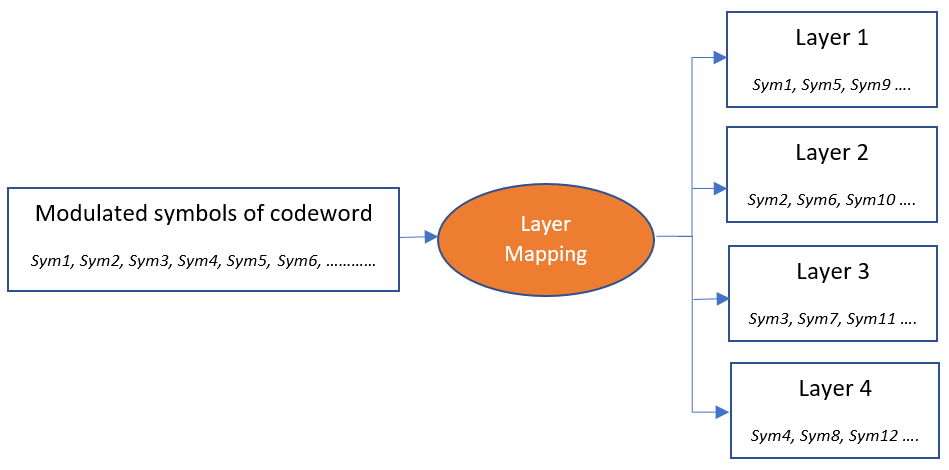Spatial multiplexing utilizes MIMO to perform multi-layer transmission. The minimum of number of transmit and receive antennas limits the number of layers (or maximum rank). The layer mapping process maps the modulated symbols of the codeword onto different layers. It maps every ${\mathit{n}}_{}^{\mathrm{th}}$ symbol of the codeword to ${\mathit{n}}_{}^{\mathrm{th}}$ layer. For instance, this figure shows the mapping of a codeword onto four layers.

Furthermore, in the DL direction, NR specification also allows two codewords and up to a maximum of 8 transmission layers. The example currently only supports single codeword for both DL and UL.

#### Precoding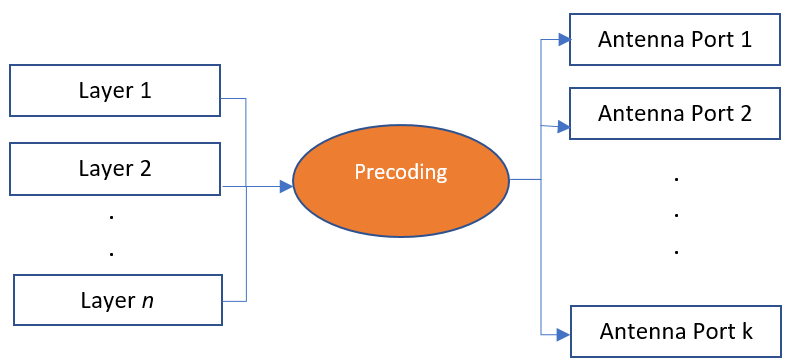Precoding, which follows the layer mapping, maps the transmission layers to antenna ports. Precoding applies a precoding matrix to the transmission layers and outputs data streams to the antenna ports.

#### Channel measurement and reporting

It consists of DL channel measurement and reporting by the UEs and UL channel measurement by gNB.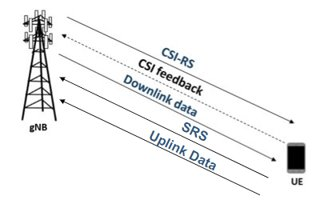#### DL channel measurement and reporting

To measure the DL channel characteristics, UEs use CSI-RS. A gNB automatically configures a full bandwidth CSI-RS resource which all the UEs in the cell use. gNB sets the CSI-RS configuration such that number of CSI-RS ports are equal to number of transmit antennas on gNB. The periodicity for CSI-RS transmission is 10 slots for FDD mode. For TDD mode, the periodicity is a multiple of the length of the DL-UL pattern (in slots). In this case, the periodicity is the least value greater than or equal to 10 slots.

CSI reporting is the process by which a UE, for DL transmissions, advises a suitable number of transmission layers (rank), PMI, and CQI values to the gNB. The UE estimates these values by performing channel measurements on its configured CSI-RS resources. For more details, see the 5G NR Downlink CSI Reporting example. The gNB scheduler uses this advice to decide the number of transmission layers, precoding matrix, and modulation and coding scheme (MCS) for PDSCHs.

#### UL channel measurement

The UL channel measurements serve as an important input to the scheduler to decide the number of transmission layers, precoding matrix and MCS for PUSCHs.

For UL channel measurements, gNB reserves one symbol for the sounding reference signal (SRS) every 'N' slots, across the entire bandwidth. 'N' is 5 for FDD. For TDD, 'N' is the least value greater than or equal to 5 slots such that it is multiple of length of the DL-UL pattern in slots. The gNB sets the SRS configuration such that number of SRS ports are equal to number of transmit antennas on UE. The gNB configures the UEs to share the reservation of SRS by differing the comb offset, cyclic shift, or transmission time. The comb size and maximum cyclic shift are both assumed as 4. In this case, gNB can configure up to 16 connected UEs to transmit SRS with periodicity as 'N' slots. If more than 16 UEs connect, the scheduler increases the SRS transmission periodicity for each UE to the next higher value (a multiple of 'N') to accommodate more UEs.

### Scenario simulation

Check if the Communications Toolbox Wireless Network Simulation Library support package is installed. If the support package is not installed, MATLAB® returns an error with a link to download and install the support package.

`wirelessnetworkSupportPackageCheck`

Create a wireless network simulator.

```rng("default") % Reset the random number generator numFrameSimulation = 10; % Simulation time in terms of number of 10 ms frames networkSimulator = wirelessNetworkSimulator.init;```

Create a gNB. Specify the carrier frequency, number of transmit antennas, number of receive antennas, and receive gain of the node.

```gNB = nrGNB(CarrierFrequency=2.6e9,ChannelBandwidth=5e6,SubcarrierSpacing=15e3,... NumTransmitAntennas=16,NumReceiveAntennas=8,ReceiveGain=11);```

Create 4 UEs. Specify the name, position, number of transmit antennas, number of receive antennas, and receive gain of each UE.

```uePositions = [300 0 0; 700 0 0; 1200 0 0; 3000 0 0]; ueNames = "UE-" + (1:size(uePositions,1)); UEs = nrUE(Name=ueNames,Position=uePositions,NumTransmitAntennas=4,NumReceiveAntennas=2,ReceiveGain=11);```

Connect the UEs to gNB. Specify the RLC bearer configuration that will be used to establish RLC bearer between gNB and each UE.

```rlcBearerConfig = nrRLCBearerConfig(SNFieldLength=6,BucketSizeDuration=10); connectUE(gNB,UEs,RLCBearerConfig=rlcBearerConfig)```

Set the periodic DL and UL application traffic pattern for UEs.

```appDataRate = 40e3; % Application data rate in kilo bits per second (kbps) for ueIdx = 1:length(UEs) % Install DL application traffic on gNB for the UE dlApp = networkTrafficOnOff(GeneratePacket=true,OnTime=numFrameSimulation*10e-3,... OffTime=0,DataRate=appDataRate); addTrafficSource(gNB,dlApp,DestinationNode=UEs(ueIdx)) % Install UL application traffic on UE for the gNB ulApp = networkTrafficOnOff(GeneratePacket=true,OnTime=numFrameSimulation*10e-3,... OffTime=0,DataRate=appDataRate); addTrafficSource(UEs(ueIdx),ulApp) end```

Add gNB and UEs to the network simulator.

```addNodes(networkSimulator,gNB) addNodes(networkSimulator,UEs)```

Create a N-by-N array of link-level channels where N is the number of nodes in the cell. An element at index (i,j) contains the channel instance from node i to node j. If element at index (i,j) is empty, it indicates that there is no channel from node i to node j. Here i and j are the node IDs.

```channelConfig = struct("DelayProfile","CDL-C","DelaySpread",300e-9); channels = createCDLChannels(channelConfig,gNB,UEs);```

Create a custom channel model using `channels` and install the custom channel on the simulator. Network simulator applies the channel for a packet in transit before passing it to the receiver.

```customChannelModel = hNRCustomChannelModel(channels); addChannelModel(networkSimulator,@customChannelModel.applyChannelModel)```

Set the `enableTraces` to `true` to log the traces. If the `enableTraces` is set to `false`, then traces are not logged in the simulation. To speed up the simulation, set the `enableTraces` to `false`.

`enableTraces = true;`

Set up scheduling logger and phy logger.

```if enableTraces % Create an object for scheduler traces logging simSchedulingLogger = helperNRSchedulingLogger(numFrameSimulation,gNB,UEs); % Create an object for PHY traces logging simPhyLogger = helperNRPhyLogger(numFrameSimulation,gNB,UEs); end```

The example updates the metrics plots periodically. Set the number of updates during the simulation.

`numMetricsSteps = 10;`

Set up metric visualizer.

```metricsVisualizer = helperNRMetricsVisualizer(gNB,UEs,NumMetricsSteps=numMetricsSteps,... PlotSchedulerMetrics=true,PlotPhyMetrics=true);```

Write the logs to MAT-file. You can use these logs for post-simulation analysis.

`simulationLogFile = "simulationLogs"; % For logging the simulation traces`

Run the simulation for the specified `numFrameSimulation` frames.

```% Calculate the simulation duration (in seconds) simulationTime = numFrameSimulation * 1e-2; % Run the simulation run(networkSimulator,simulationTime);```

```gNBStats = statistics(gNB); ueStats = statistics(UEs);```

At the end of the simulation, the achieved value for system performance indicators is compared to their theoretical peak values (considering zero overheads). Performance indicators displayed are achieved data rate (UL and DL), achieved spectral efficiency (UL and DL), and block error rate (BLER) observed for UEs (UL and DL). The peak values are calculated as per 3GPP TR 37.910.

`displayPerformanceIndicators(metricsVisualizer)`
```Peak UL Throughput: 124.42 Mbps. Achieved Cell UL Throughput: 26.71 Mbps Achieved UL Throughput for each UE: [6.76 6.72 6.78 6.45] Peak UL spectral efficiency: 24.88 bits/s/Hz. Achieved UL spectral efficiency for cell: 5.34 bits/s/Hz Block error rate for each UE in the uplink direction: [0 0 0 0] Peak DL Throughput: 62.21 Mbps. Achieved Cell DL Throughput: 43.66 Mbps Achieved DL Throughput for each UE: [12.47 12.13 12.37 6.69] Peak DL spectral efficiency: 12.44 bits/s/Hz. Achieved DL spectral efficiency for cell: 8.73 bits/s/Hz Block error rate for each UE in the downlink direction: [0 0 0 0] ```

### Simulation Visualization

The run-time visualization shown are:

• Display of UL scheduling metrics plots: The Uplink Scheduler Performance Metrics figure includes these plots: UL transmission rate (per UE and cell), UL throughput (per UE and cell), resource share percentage among UEs (out of the total UL resources) to convey the fairness of scheduling, and pending UL buffer status of the UEs to show whether UEs are getting sufficient resources. To show the maximum achievable data rate value for UL throughput, this example uses a dashed line in the throughput and transmission rate plots. The UL transmission rate plot shows the rate of MAC data transmission for each UE and across the cell. The calculation includes transport block size (TBS) of new transmissions as well as the TBS of retransmissions. The units are in megabits per second (Mbps). The throughput plot shows the rate (in Mbps) of successful MAC data reception at gNB from each UE and across the cell.

• Display of DL scheduling metrics plots: The Downlink Scheduler Performance Metrics figure includes these plots: DL transmission rate (per UE and cell), DL throughput (per UE and cell), resource share percentage among UEs (out of the total DL resources) to convey the fairness of scheduling, and pending DL buffer status of the UEs to show whether UEs are getting sufficient resources. To show the maximum achievable data rate value for DL throughput, this example uses a dashed line in the throughput and transmission rate plots. The DL transmission rate plot shows the rate (in Mbps) of MAC data transmission for each UE and across the cell. The calculation includes TBS of new transmissions as well as the TBS of retransmissions. The throughput plot shows the rate (in Mbps) of successful MAC data reception at each UE and across the cell.

• Display of DL and UL Block Error Rates: The two sub-plots displayed in 'Block Error Rate (BLER) Visualization' shows the BLER (for each UE) observed in the uplink and downlink directions, as the simulation progresses.

### Simulation Logs

The simulation logs are saved in MAT-files for post-simulation analysis. The per time step logs, scheduling assignment logs, and Phy reception logs are saved in the MAT-file `simulationLogFile`. After the simulation, open the file to load `DLTimeStepLogs`, `ULTimeStepLogs`, `SchedulingAssignmentLogs`, `PhyReceptionLogs` in the workspace.

Time step logs: Both the DL and UL time step logs follow the same format. The table shows sample time step entries.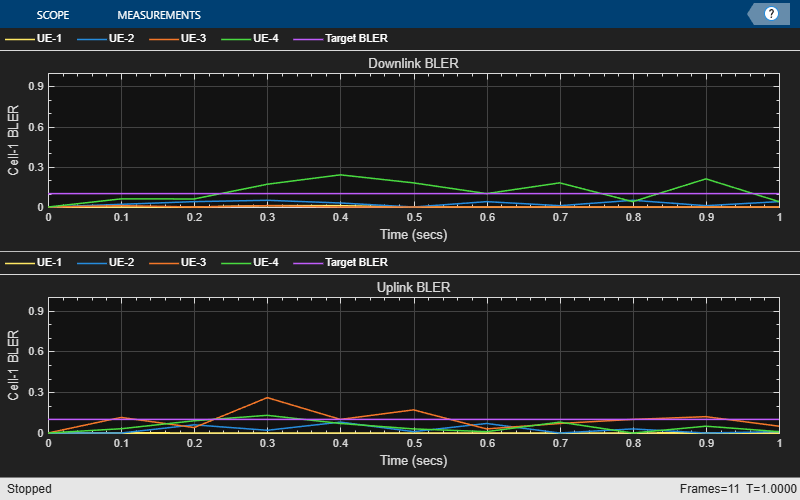Each row of the table represents a slot and contains the following information:

• Timestamp: Time (in milliseconds) since the start of simulation.

• Frame: Frame number.

• Slot: Slot number in the frame.

• Frequency Allocation: N-by-P matrix. N is the number of UEs. For frequency resource allocation type-1, P is 2. The first element of frequency resource allocation indicates the starting RB. The second element indicates the number of RBs allocated. For example, [0,6;6,6;12,6;18,7] indicates this: the scheduler has assigned 6 RBs (starting from 0) to UE-1, 6 RBs (starting from 6) to UE-2, 6 RBs (starting from 12) to UE-3, and 7 RBs (starting from 18) to UE-4. For frequency resource allocation type-0, P is the number of resource block groups (RBGs). The scheduler assigns an RBG to a particular UE by setting the corresponding bit to 1. For example, [ 0 0 1 1 0 1 0 1 0 1 0 0 0; 1 1 0 0 0 0 0 0 0 0 1 0 0; 0 0 0 0 1 0 1 0 1 0 0 1 1; 0 0 0 0 0 0 0 0 0 0 0 0 0] indicates that the bandwidth has 13 RBGs. The scheduler has assigned the RBG indices 2, 3, 5, 7, and 9 to UE-1, the RBG indices 0, 1, and 10 to UE-2, and the RBG indices 4, 6, 8, 11, and 12 to UE-3. The scheduler has not assigned any RBGs to UE-4.

• MCS: Row vector of length N, where N is the number of UEs. Each value corresponds to the modulation and coding scheme (MCS) index for the transmission. For example, [10 12 8 -1] indicates these: a) the scheduler has assigned the resources to UE-1, UE-2, and UE-3 for this slot and b) UE-1, UE-2 AND UE-3 use the MCS values 10, 12, and 8, respectively. The MCS index corresponds to 3GPP TS 38.214 - Table 5.1.3.1-2.

• HARQ Process: Row vector of length N, where N is the number of UEs. The value is the HARQ process ID used by UE for the transmission. For example, [0 3 6 -1] indicates these: a) the scheduler has assigned the resources to UE-1, UE-2, and UE-3 for this slot and b) UE-1, UE-2 AND UE-3 use the HARQ process IDs 0, 3, and 6, respectively.

• NDI: Row vector of length N, where N is the number of UEs. The value is the new data indicator (NDI) flag value in the assignment for transmission. For example, [0 0 1 -1] indicates these: a) The scheduler has assigned the resources to UE-1, UE-2, and UE-3 for this slot and b) UE-1, UE-2, and UE-3 use the NDI flag values 0, 0, and 1, respectively. The NDI flag determines whether the UEs have used a new transmission or a retransmission.

• Tx Type: Row vector of length N, where N is the number of UEs. Tx Type specifies the transmission type (new transmission or retransmission), specified as one of these values: '`newTx`', '`reTx`', or '`noTx`'. '`noTx`' indicates that the scheduler has not allocated the resources to the UE. For example, ['`newTx`' '`newTx`' '`reTx`' '`noTx`'] indicates that the scheduler has assigned the resources to UE-1, UE-2, and UE-3 for this slot. UE-1 and UE-2 transmit a new packet from the specified HARQ process while UE-3 retransmits the packet in the buffer of the specified HARQ process.

• Channel Quality: Cell array of length N, where N is the number of UEs. An element at index i contains the channel quality information for UE with RNTI i.

• HARQ NDI Status: N-by-P matrix, where N is the number of UEs and P is the number of HARQ processes on UEs. A matrix element at position (i, j) is the last received NDI flag at UE i for HARQ process ID j. For new transmissions, this value and the NDI flag in the assignment must toggle. For example, in slot 4 of frame 0 described in the scheduling log, the last NDI flag value for HARQ ID 3 at UE-1 is 1. To indicate a new transmission, the NDI flag value changes to 0.

• Transmitted Bytes: Row vector of length N, where N is the number of UEs. The values represent MAC bytes transmitted per UE in this slot. The value only includes new transmission bytes. For retransmissions, the value is zero.

• Buffer Status of UEs: Row vector of length N, where N is the number of UEs. The values represent the amount of pending buffer per UE.

Scheduling assignment logs: A log of all the scheduling assignments and related information. The table shows sample log entries.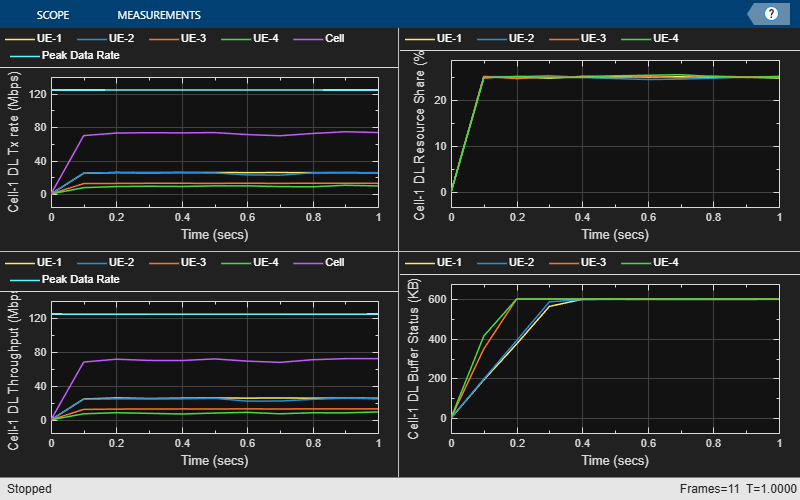Each row of the table represents a DL or UL allocation grant and contains these information:

• RNTI: Radio network temporary identifier of UE.

• Frame: Frame number.

• Slot: Slot number in the frame.

• Grant Type: Grant type as 'DL' or 'UL'.

• Frequency Allocation: Row vector of length P. For frequency resource allocation type-1, P is 2. For frequency resource allocation type-0, P is the number of RBGs.

• Start Symbol: Start symbol for the transmission.

• Num Symbols: Number of symbols for the transmission.

• MCS: Modulation and coding scheme (MCS) index for the transmission.

• NumLayers: Number of spatial layers for the transmission.

• HARQ Process: HARQ process ID used for the transmission.

• NDI: NDI flag value for transmission.

• Tx Type: Transmission type, specified as one of these values: '`newTx`', '`reTx`'.

• Feedback Slot Offset (DL grants only): Slot offset for the feedback of PDSCH transmission.

• Channel Quality: Row vector of length P, where P is the number of RBs in the bandwidth. An element at position j corresponds to the CQI value for UE at RB j.

Phy reception logs: A log of Phy packet reception information observed in the uplink and downlink directions. This table shows the sample log entries.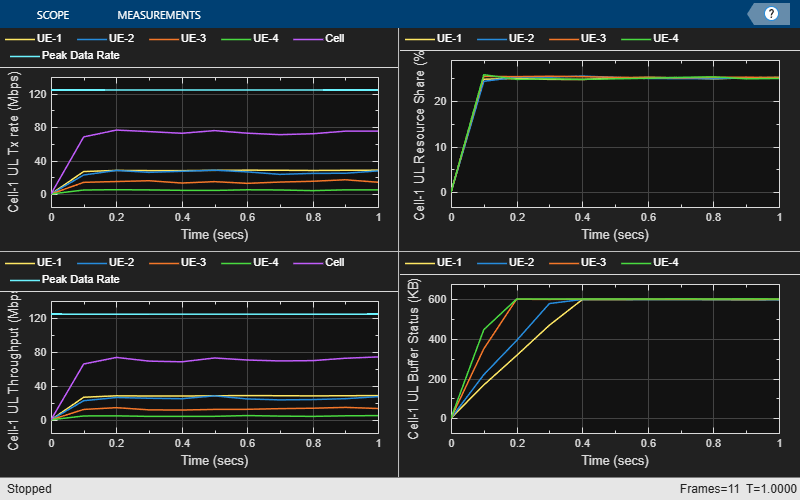Each row of the table represents a slot and contains the following information:

• Timestamp: Time (in milliseconds) since the start of simulation.

• Frame: Frame number.

• Slot: Slot number in the frame.

• Symbol: Symbol number in the slot.

• Number of Decode Failures (DL): Row vector of length N, where N is the number of UEs. The values represent the number of decode failures at UEs in this slot.

• Number of Packets (DL): Row vector of length N, where N is the number of UEs. The values represent the number of packets received at UEs in this slot.

• Number of Decode Failures (UL): Row vector of length N, where N is the number of UEs. The values represent the number of decode failures at gNB for each UE in this slot.

• Number of Packets (UL): Row vector of length N, where N is the number of UEs. The values represent the number of packets received at gNB for each UE in this slot.

Save the simulation logs in a MAT file.

```if enableTraces simulationLogs = cell(1,1); if gNB.DuplexMode == "FDD" logInfo = struct("DLTimeStepLogs",[],"ULTimeStepLogs",[],... "SchedulingAssignmentLogs",[],"PhyReceptionLogs",[]); [logInfo.DLTimeStepLogs,logInfo.ULTimeStepLogs] = getSchedulingLogs(simSchedulingLogger); else % TDD logInfo = struct("TimeStepLogs",[],"SchedulingAssignmentLogs",[],"PhyReceptionLogs",[]); logInfo.TimeStepLogs = getSchedulingLogs(simSchedulingLogger); end % Get the scheduling assignments log logInfo.SchedulingAssignmentLogs = getGrantLogs(simSchedulingLogger); % Get the Phy reception logs logInfo.PhyReceptionLogs = getReceptionLogs(simPhyLogger); % Save simulation logs in a MAT-file simulationLogs{1} = logInfo; save(simulationLogFile,"simulationLogs") end```

### Further Exploration

#### Importing ray-traces

You can modify this example to customize the CDL channel model parameters by using the output of a ray tracing analysis. Refer to the CellPerformanceWithRayTrace.m script, which demonstrates this workflow. The script follows the CDL Channel Model Customization with Ray Tracing example to configure the 'Custom' delay profile of nrCDLChannel object.

### Local functions

Set up CDL channel instances for the cell. For DL, set up a CDL channel instance from the gNB to each UE. For UL, set up a CDL channel instance from each UE to gNB.

```function channels = createCDLChannels(channelConfig,gNB,UEs) %createCDLChannels Create channels between gNB and UEs in a cell % CHANNELS = createCDLChannels(CHANNELCONFIG,GNB,UES) creates channels % between GNB and UES in a cell. % % CHANNELS is a N-by-N array where N is the number of nodes in the cell. % % CHANNLECONFIG is a struct with these fields - DelayProfile and % DelaySpread. % % GNB is an nrGNB object. % % UES is an array of nrUE objects. numUEs = length(UEs); numNodes = length(gNB) + numUEs; % Create channel matrix to hold the channel objects channels = cell(numNodes,numNodes); % Get the sample rate of waveform waveformInfo = nrOFDMInfo(gNB.NumResourceBlocks,gNB.SubcarrierSpacing/1e3); sampleRate = waveformInfo.SampleRate; for ueIdx = 1:numUEs % Configure the uplink channel model between gNB and UE channel = nrCDLChannel; channel.DelayProfile = channelConfig.DelayProfile; channel.DelaySpread = channelConfig.DelaySpread; channel.Seed = 73 + (ueIdx - 1); channel.CarrierFrequency = gNB.CarrierFrequency; channel = hArrayGeometry(channel, UEs(ueIdx).NumTransmitAntennas,gNB.NumReceiveAntennas,... "uplink"); channel.SampleRate = sampleRate; channel.ChannelFiltering = false; channels{UEs(ueIdx).ID, gNB.ID} = channel; % Configure the downlink channel model between gNB and UE channel = nrCDLChannel; channel.DelayProfile = channelConfig.DelayProfile; channel.DelaySpread = channelConfig.DelaySpread; channel.Seed = 73 + (ueIdx - 1); channel.CarrierFrequency = gNB.CarrierFrequency; channel = hArrayGeometry(channel, gNB.NumTransmitAntennas,UEs(ueIdx).NumReceiveAntennas,... "downlink"); channel.SampleRate = sampleRate; channel.ChannelFiltering = false; channels{gNB.ID, UEs(ueIdx).ID} = channel; end end```

### Appendix

The example uses these helper classes:

 3GPP TS 38.104. “NR; Base Station (BS) radio transmission and reception.” 3rd Generation Partnership Project; Technical Specification Group Radio Access Network.

 3GPP TS 38.214. “NR; Physical layer procedures for data.” 3rd Generation Partnership Project; Technical Specification Group Radio Access Network.

 3GPP TS 38.321. “NR; Medium Access Control (MAC) protocol specification.” 3rd Generation Partnership Project; Technical Specification Group Radio Access Network.

 3GPP TS 38.322. “NR; Radio Link Control (RLC) protocol specification.” 3rd Generation Partnership Project; Technical Specification Group Radio Access Network.

 3GPP TS 38.323. “NR; Packet Data Convergence Protocol (PDCP) specification.” 3rd Generation Partnership Project; Technical Specification Group Radio Access Network.

 3GPP TS 38.331. “NR; Radio Resource Control (RRC) protocol specification.” 3rd Generation Partnership Project; Technical Specification Group Radio Access Network.

 3GPP TR 37.910. “Study on self evaluation towards IMT-2020 submission.” 3rd Generation Partnership Project; Technical Specification Group Radio Access Network.